# Matplotlib – An Intro to Creating Graphs with Python

Data visualizations are an important method of sharing your data with others. Some people refer to visualizations as plots, charts, or graphs. These names are synonymous in this article.

Python has many 3rd party packages that do data visualizations. In fact, there are so many that it can be somewhat overwhelming. One of the oldest and most popular is Matplotlib. Matplotlib is known for creating static, animated, and interactive visualizations in Python.

You can create many different types of plots and charts with Matplotlib. It also integrates well with other data science and math libraries like NumPy and pandas. You will also find that Matplotlib works with most of Python’s GUI toolkits, such as Tkinter, wxPython and PyQt. Because Matplotlib is so well known, it will be the graphing package that is covered in this article.

You will be learning about the following topics:

• Creating a Simple Line Chart with PyPlot
• Creating a Bar Chart
• Creating a Pie Chart
• Creating a Legend
• Showing Multiple Figures

Let’s start plotting with Matplotlib!

## Installing Matplotlib

You will need to install Matplotlib to be able to use it. Fortunately, that is easy to do with `pip`:

``````python -m pip install matplotlib
``````

This will install Matplotlib as well as any dependencies that it requires. Now you are ready to start graphing!

## Creating a Simple Line Chart with PyPlot

Creating charts (or plots) is the primary purpose of using a plotting package. Matplotlib has a sub-module called `pyplot` that you will be using to create a chart. To get started, go ahead and create a new file named `line_plot.py` and add the following code:

```# line_plot.py

import matplotlib.pyplot as plt

def line_plot(numbers):
plt.plot(numbers)
plt.ylabel('Random numbers')
plt.show()

if __name__ == '__main__':
numbers = [2, 4, 1, 6]
line_plot(numbers)
```

Here you import `matplotlib.pyplot` as `plt`. Then you create a `line_plot()` which takes in a Python list of numbers. To plot the numbers, you use the `plot()` function. You also add a label to the y-axis. Finally, you call `show()` to display the plot.

You should now see a window that looks like this:Now you know how to create a simple line chart using Matplotlib! Now you will find out how to make a bar chart in the next section.

## Creating a Bar Chart

Creating a bar chart with Matplotlib is very similar to how you created a line plot. It just takes a few extra arguments. Go ahead and create a new file named `bar_chart.py` and enter the following code into it:

```# bar_chart.py

import matplotlib.pyplot as plt

def bar_chart(numbers, labels, pos):
plt.bar(pos, numbers, color='blue')
plt.xticks(ticks=pos, labels=labels)
plt.show()

if __name__ == '__main__':
numbers = [2, 1, 4, 6]
labels = ['Electric', 'Solar', 'Diesel', 'Unleaded']
pos = list(range(4))
bar_chart(numbers, labels, pos)
```

When you create a bar chart using `bar()`, you pass in a list of values for the x-axis. Then you pass in a list of heights for the bars. You can also optionally set a color for the bars. In this case, you set them to “blue”. Next, you set the `xticks()`, which are the tick marks that should appear along the x-axis. You also pass in a list of labels that correspond to the ticks.

Go ahead and run this code and you should see the following graph:You can also make a horizontal bar chart with Matplotlib. All you need to do is change `bar()` to `barh()`. Create a new file named `bar_chartsh.py` and add this code:

```# bar_charth.py

import matplotlib.pyplot as plt

def bar_charth(numbers, labels, pos):
plt.barh(pos, numbers, color='blue')
plt.yticks(ticks=pos, labels=labels)
plt.show()

if __name__ == '__main__':
numbers = [2, 1, 4, 6]
labels = ['Electric', 'Solar', 'Diesel', 'Unleaded']
pos = list(range(4))
bar_charth(numbers, labels, pos)
```

There is one other sneaky change here. Can you spot it? The change is that since it is now a horizontal bar chart, you will want to set the `yticks()` instead of the `xticks()` or it won’t look quite right.

Once you have it all ready to go, run the code and you will see the following: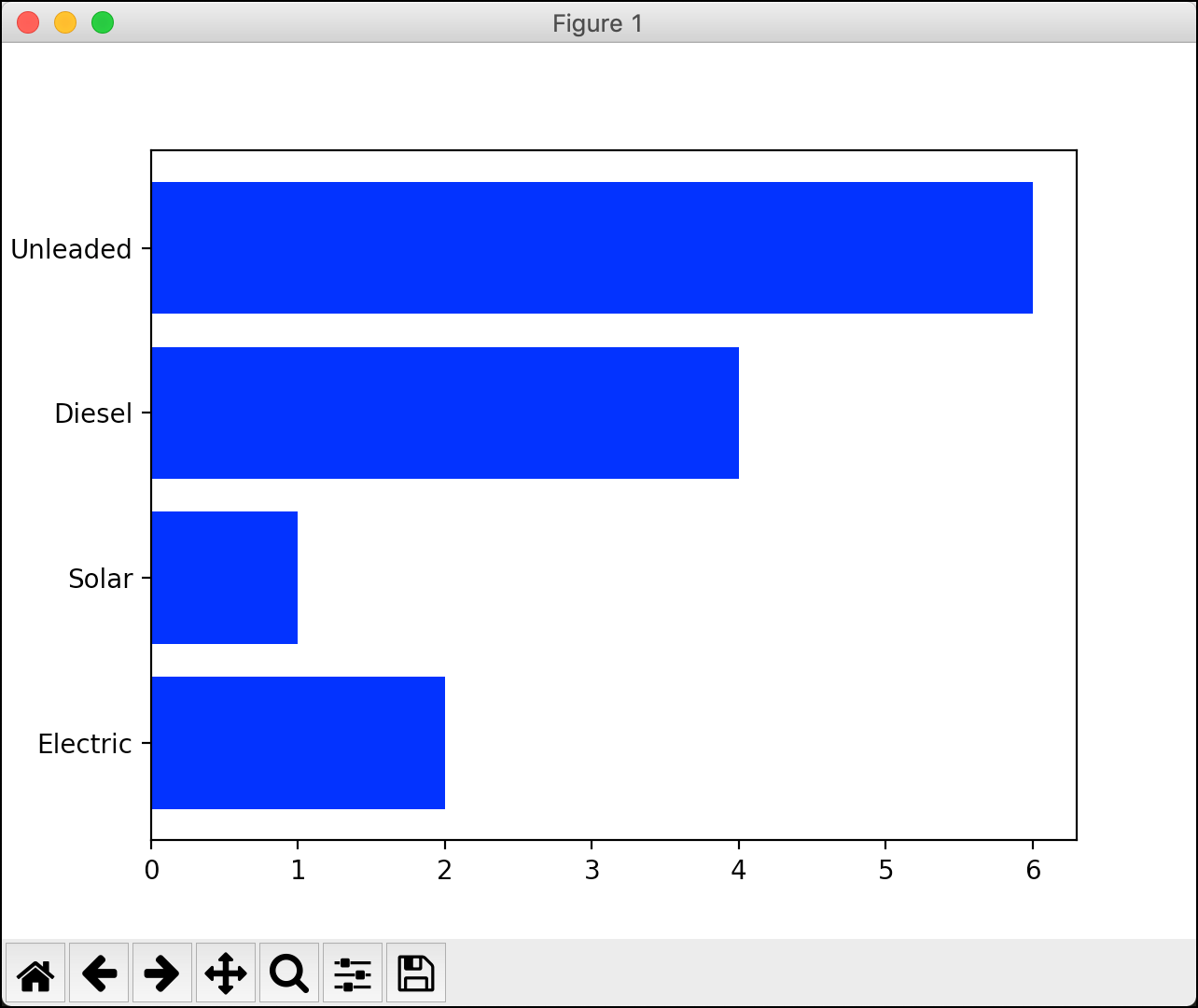That looks great and it didn’t take very much code at all! Now let’s find out how to create a pie chart with Matplotlib.

## Creating a Pie Chart

Pie charts are a bit of a different beast. To create a pie chart, you will be using Matplotlib’s `subplots()` function, which returns a `Figure` and an `Axes` object. To see how that works, create a new file named `pie_chart_plain.py` and put this code in it:

```# pie_chart_plain.py

import matplotlib.pyplot as plt

def pie_chart():
numbers = [40, 35, 15, 10]
labels = ['Python', 'Ruby', 'C++', 'PHP']

fig1, ax1 = plt.subplots()
ax1.pie(numbers, labels=labels)
plt.show()

if __name__ == '__main__':
pie_chart()
```

In this code, you create `subplots()` and then use the `pie()` method of the `Axes` object. You pass in a list of numbers as you did before, as well as a list of labels. Then when you run the code, you will see your pie chart: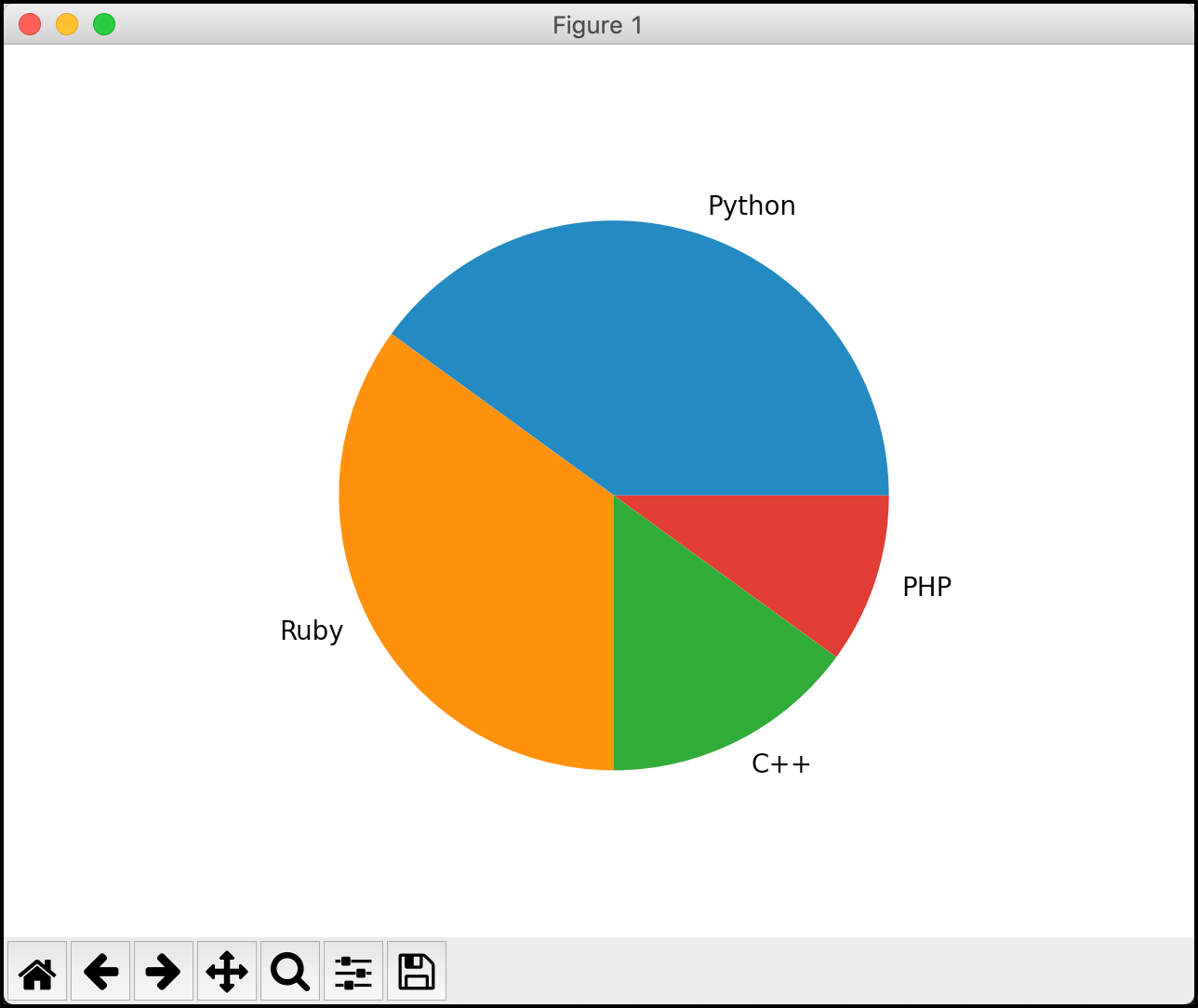That’s pretty nice for such a short piece of code. But you can make your pie charts look even better. Create a new file named `pie_chart_fancy.py` and add this code to see how:

```# pie_chart_fancy.py

import matplotlib.pyplot as plt

def pie_chart():
numbers = [40, 35, 15, 10]
labels = ['Python', 'Ruby', 'C++', 'PHP']
# Explode the first slice (Python)
explode = (0.1, 0, 0, 0)

fig1, ax1 = plt.subplots()
ax1.pie(numbers, explode=explode, labels=labels,
autopct='%1.1f%%')
ax1.axis('equal')
plt.show()

if __name__ == '__main__':
pie_chart()
```

For this example, you use the `explode` parameter to tell the pie chart to “explode” or remove a slice from the pie. In this case, you remove the first slice, which corresponds to “Python”. You also add a `shadow` to the pie chart. You can tell your pie chart to rotate a certain number of degrees counter-clockwise by setting the `startangle`. If you’d like to show the slice percentages, you can use `autopct`, which will use Python’s string interpolation syntax.

When you run this code, your pie chart will now look like this: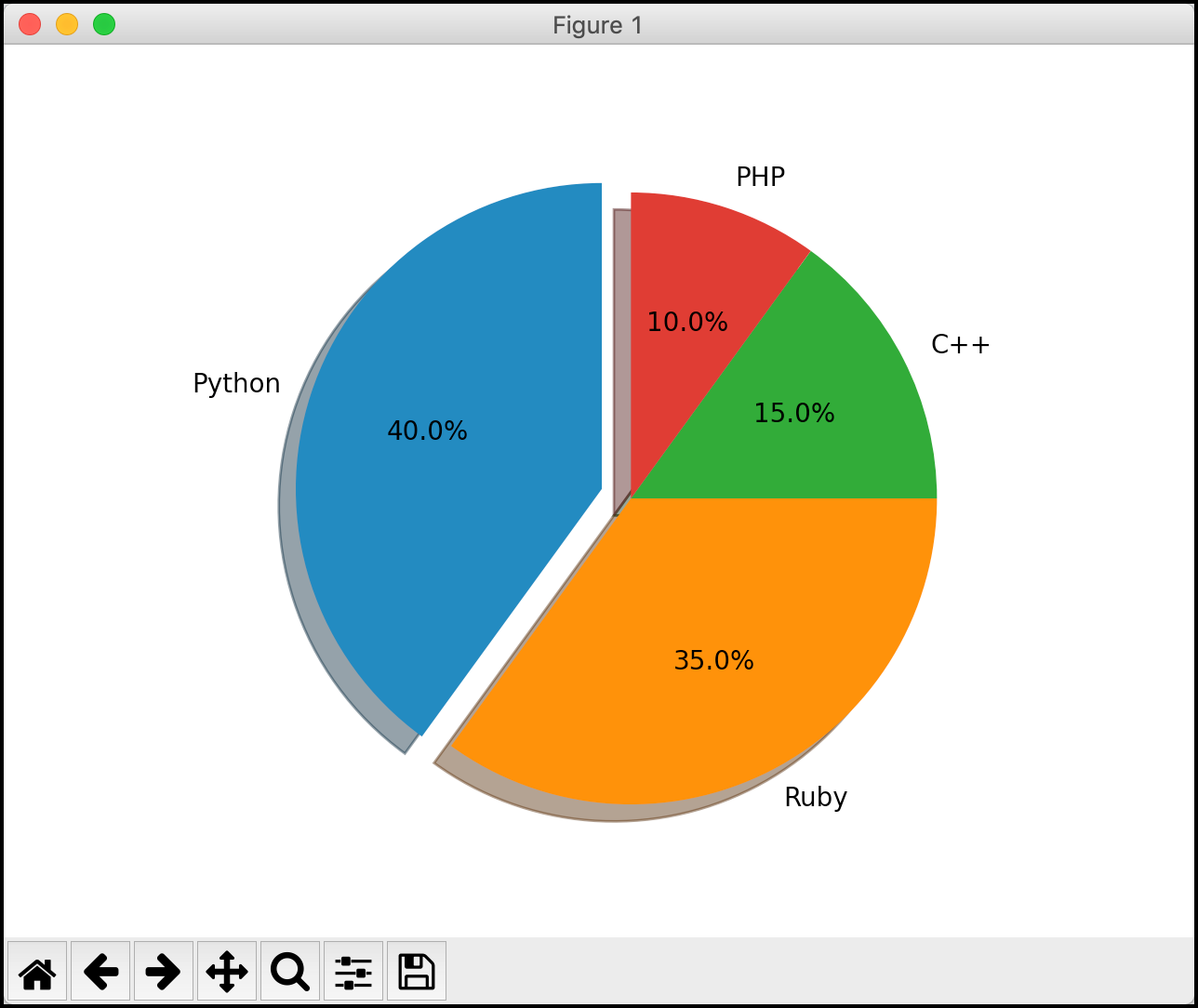Isn’t that neat? Your pie chart now looks much more polished! Now it’s time to learn how to add labels to your other graphs!

When you are graphing data, you will usually want to label the axes. You can label the x-axis by using the `xlabel()` function and you can label the y-axis by using the corresponding `ylabel()` function. To see how this works, create a file named `bar_chart_labels.py` and add this code to it:

```# bar_chart_labels.py

import matplotlib.pyplot as plt

def bar_chart(numbers, labels, pos):
plt.bar(pos, numbers, color='blue')
plt.xticks(ticks=pos, labels=labels)
plt.xlabel('Vehicle Types')
plt.ylabel('Number of Vehicles')
plt.show()

if __name__ == '__main__':
numbers = [2, 1, 4, 6]
labels = ['Electric', 'Solar', 'Diesel', 'Unleaded']
pos = list(range(4))
bar_chart(numbers, labels, pos)
```

Here you call both `xlabel()` and `ylabel()` and set them to different strings. This adds some explanatory text underneath the graph and to the left of the graph, respectively. Here is what the result looks like: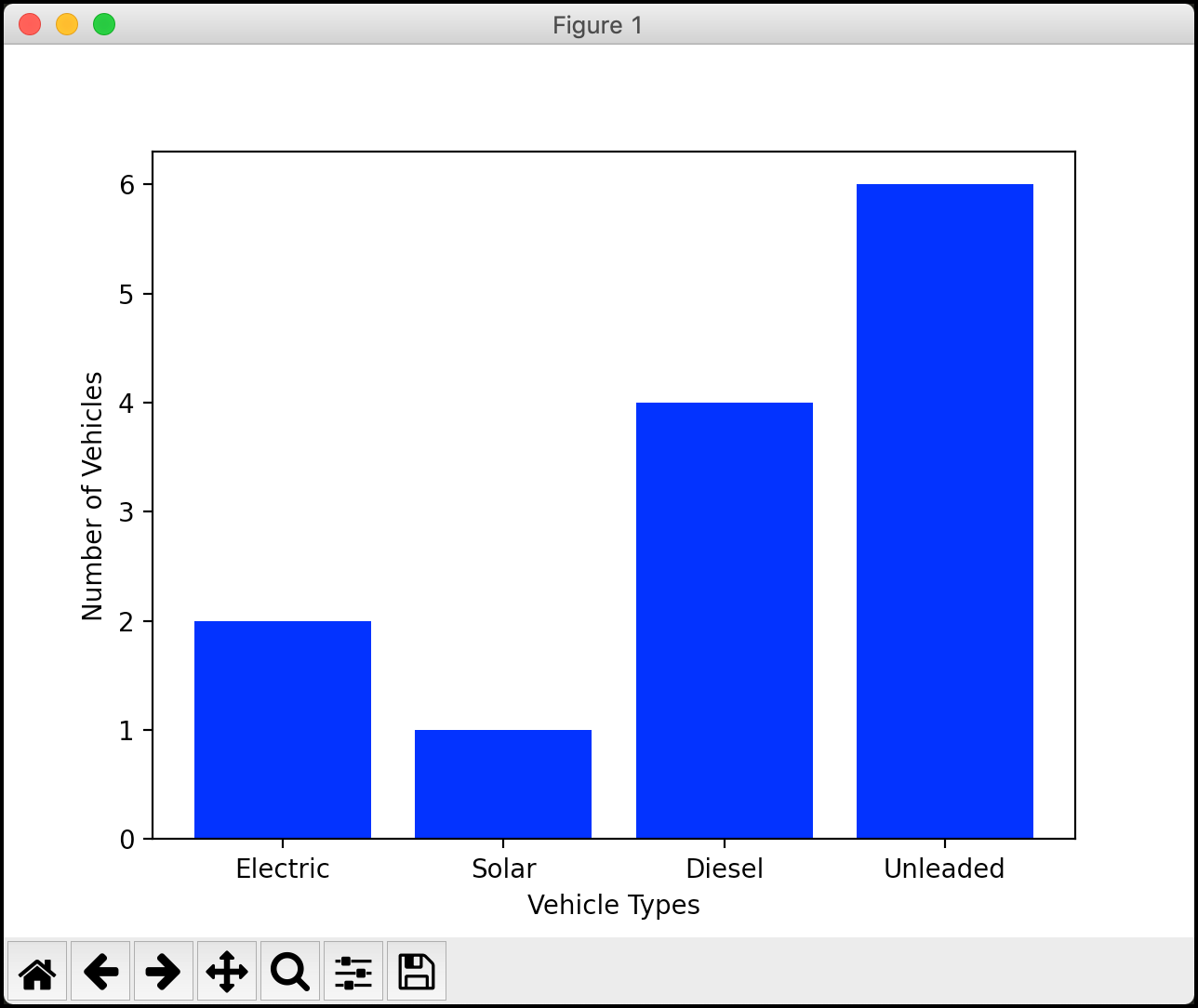That looks quite nice. Your graph is easier to understand, but it is missing a title. You will learn how to do that in the next section!

Adding titles to your graphs with Matplotlib is quite straightforward. In fact, all you need to do is use the `title()` function to add one. To find out how, create a new file named `bar_chart_title.py` and add this code to it:

```# bar_chart_title.py

import matplotlib.pyplot as plt

def bar_chart(numbers, labels, pos):
plt.bar(pos, [4, 5, 6, 3], color='green')
plt.bar(pos, numbers, color='blue')
plt.xticks(ticks=pos, labels=labels)
plt.title('Gas Used in Various Vehicles')
plt.xlabel('Vehicle Types')
plt.ylabel('Number of Vehicles')
plt.show()

if __name__ == '__main__':
numbers = [2, 1, 4, 6]
labels = ['Electric', 'Solar', 'Diesel', 'Unleaded']
pos = list(range(4))
bar_chart(numbers, labels, pos)
```

The primary change here is on line 9 where you call `title()` and pass in a string. This sets the title for the graph and centers it along the top by default. You can change the location slightly by setting the `loc` parameter to “left” or “right”, but you can’t specify that the title be anywhere but the top. There is also a `fontdict` parameter that you can use for controlling the appearance of the title font.

You also add a new bar plot to the graph. This helps you see what a stacked bar plot looks like and also prepares you for the next section.

Here is what your graph looks like now: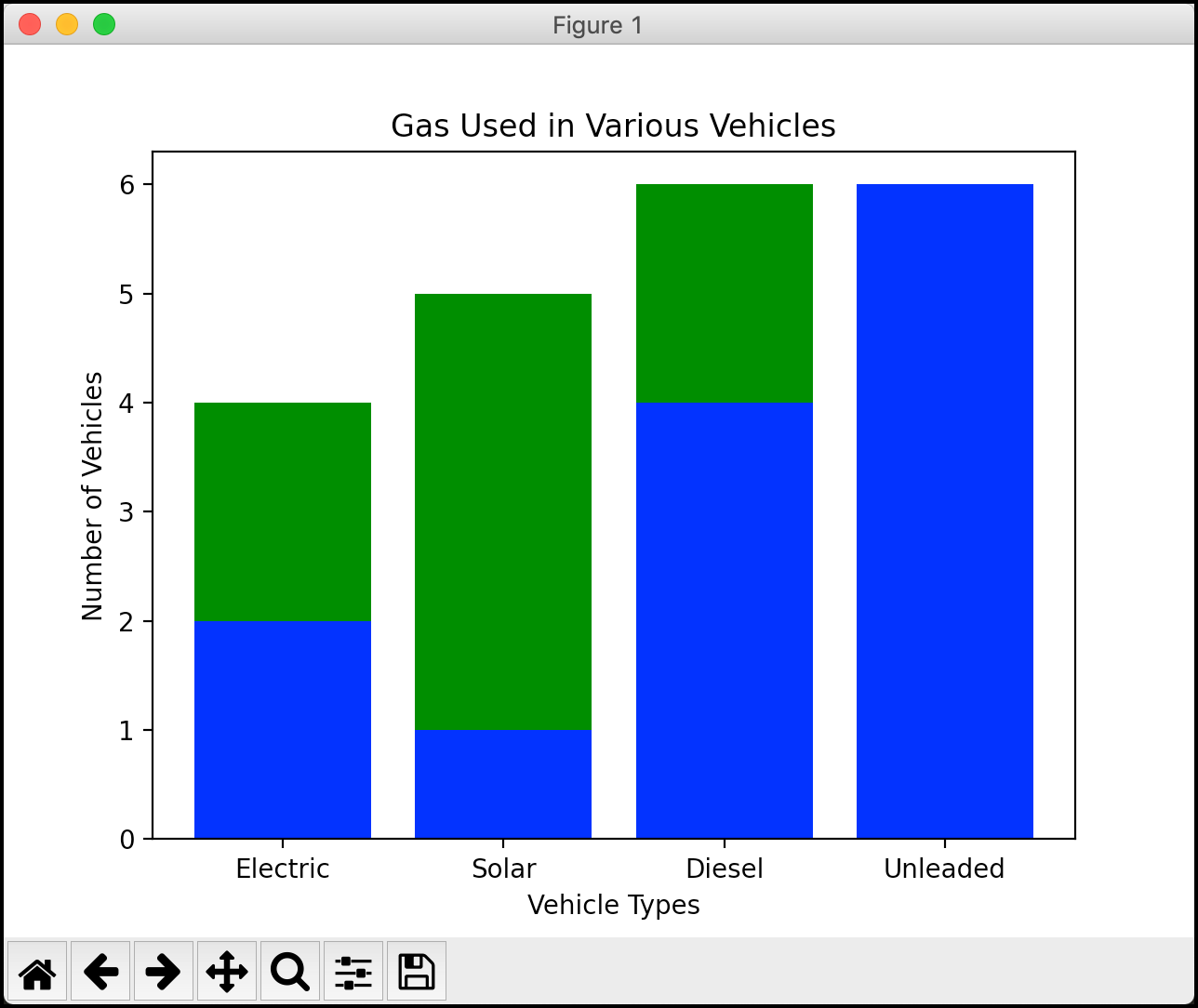This graph looks even better, but it’s still missing something. Oh! You need a legend! Let’s find out how to do that next.

## Creating a Legend

Adding a legend to your Matplotlib graph is also straightforward. You will use the `legend()` function to add one. Create a new file named `bar_chart_legend.py`. Then, add this code to it:

```# bar_chart_legend.py

import matplotlib.pyplot as plt

def bar_chart(numbers, labels, pos):
plt.bar(pos, [4, 5, 6, 3], color='green')
plt.bar(pos, numbers, color='blue')
plt.xticks(ticks=pos, labels=labels)
plt.xlabel('Vehicle Types')
plt.ylabel('Number of Vehicles')
plt.legend(['First Label', 'Second Label'], loc='upper left')
plt.show()

if __name__ == '__main__':
numbers = [2, 1, 4, 6]
labels = ['Electric', 'Solar', 'Diesel', 'Unleaded']
pos = list(range(4))
bar_chart(numbers, labels, pos)
```

Here you add a `legend()` right before you `show()` the graph. When you create a legend, you can set the labels by passing in a list of strings. The list should match the number of plots in your graph. You can also set the location of the legend by using the `loc` parameter.

When you run this code, you will see your graph updated to look like this: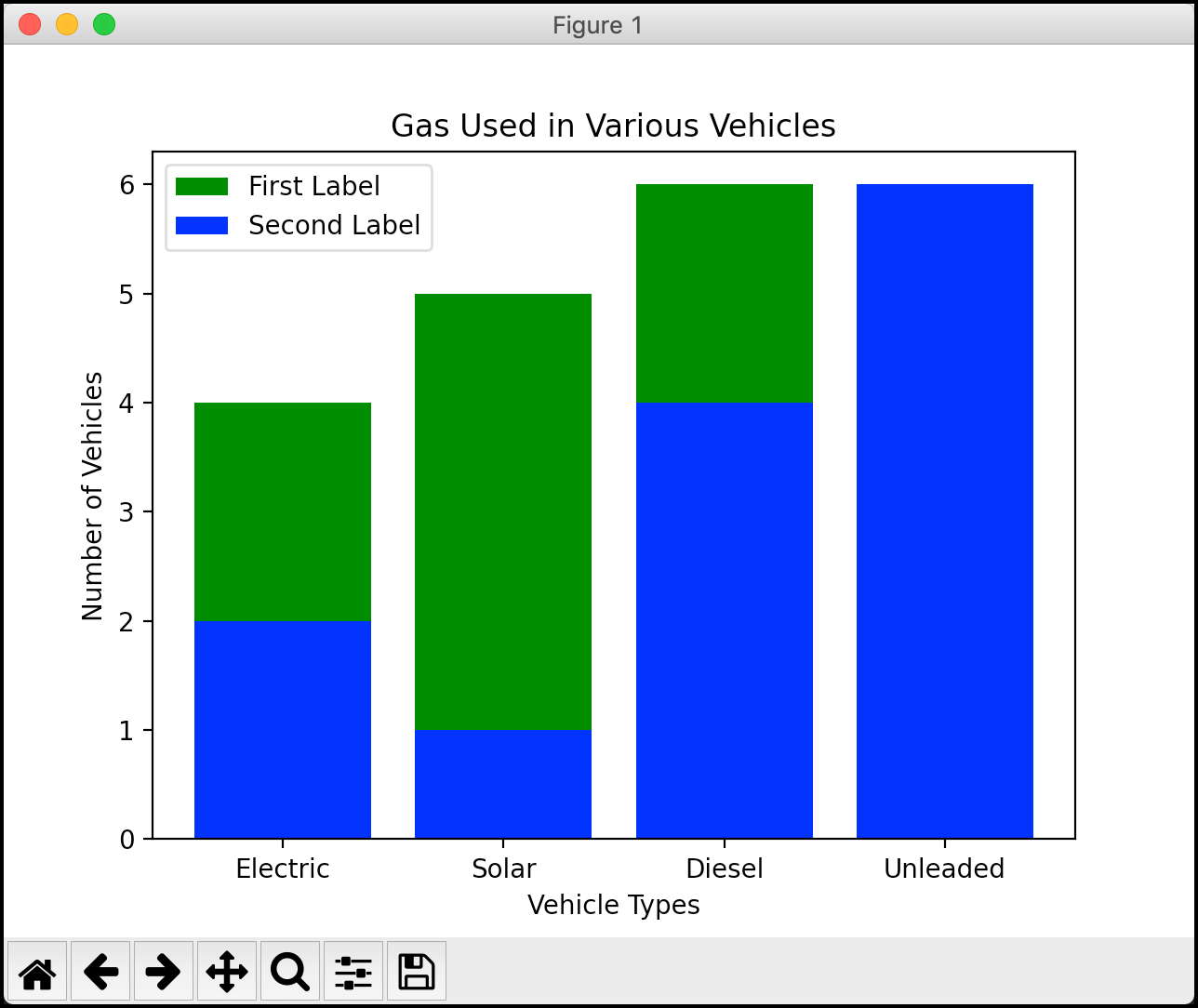Now your graph has all the normal components that you would expect to have in a graph. At this point you’ve already seen a lot of tasks you can accomplish using Matplotlib. The last topic to learn about is how to add multiple figures with Matplotlib.

## Showing Multiple Figures

Matplotlib allows you to create several plots before you show them. This lets you work with multiple datasets at once. There are several different ways you can do this. You will look at one of the simplest ways to do so.

Create a new file named `multiple_figures.py` and add this code:

```# multiple_figures.py

import matplotlib.pyplot as plt

def line_plot(numbers, numbers2):
first_plot = plt.figure(1)
plt.plot(numbers)

second_plot = plt.figure(2)
plt.plot(numbers2)
plt.show()

if __name__ == '__main__':
numbers = [2, 4, 1, 6]
more_numbers = [5, 1, 10, 3]
line_plot(numbers, more_numbers)
```

Here you create two line plots. Before you plot, you call `figure()`, which creates a top-level container for the plots that follow after it is called. Thus the first plot is added to figure one and the second plot it added to figure 2. When you then call `show()` at the end, Matplotlib will open two windows with each graph shown separately.

Run the code and you will see the following two windows on your machine: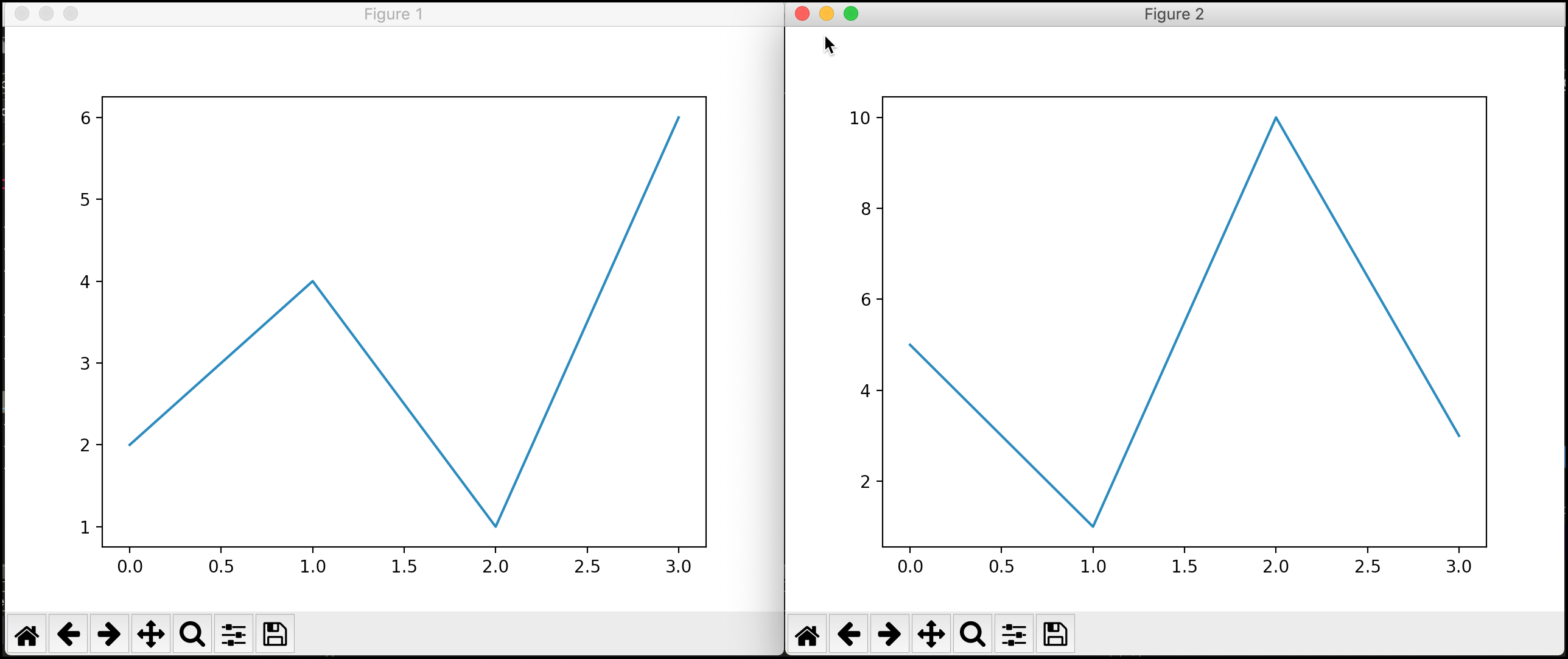Matplotlib also supports adding two or more plots to a single window. To see how that works, create another new file and name this one `multiple_plots.py`. To make things more interesting, you will use NumPy in this example to create the two plots.

Note: If you haven’t already, you will need to install NumPy to get this example to work.

This example is based on one from the Matplotlib documentation:

```# multiple_plots.py

import matplotlib.pyplot as plt
import numpy as np

def multiple_plots():
# Some example data to display
x = np.linspace(0, 2 * np.pi, 400)
y = np.sin(x ** 2)

fig, axs = plt.subplots(2)
fig.suptitle('Vertically stacked subplots')
axs.plot(x, y)
axs.plot(x, -y)
plt.show()

if __name__ == '__main__':
multiple_plots()
```

Here you create what amounts to two separate sine wave graphs. To make them both show up in the same window, you use a call to `subplots()`, which is a handy utility for creating multiple figures in a single call. You can then use the `Axes` object that it returns to plot the data you created with NumPy.

The result ends up looking like this: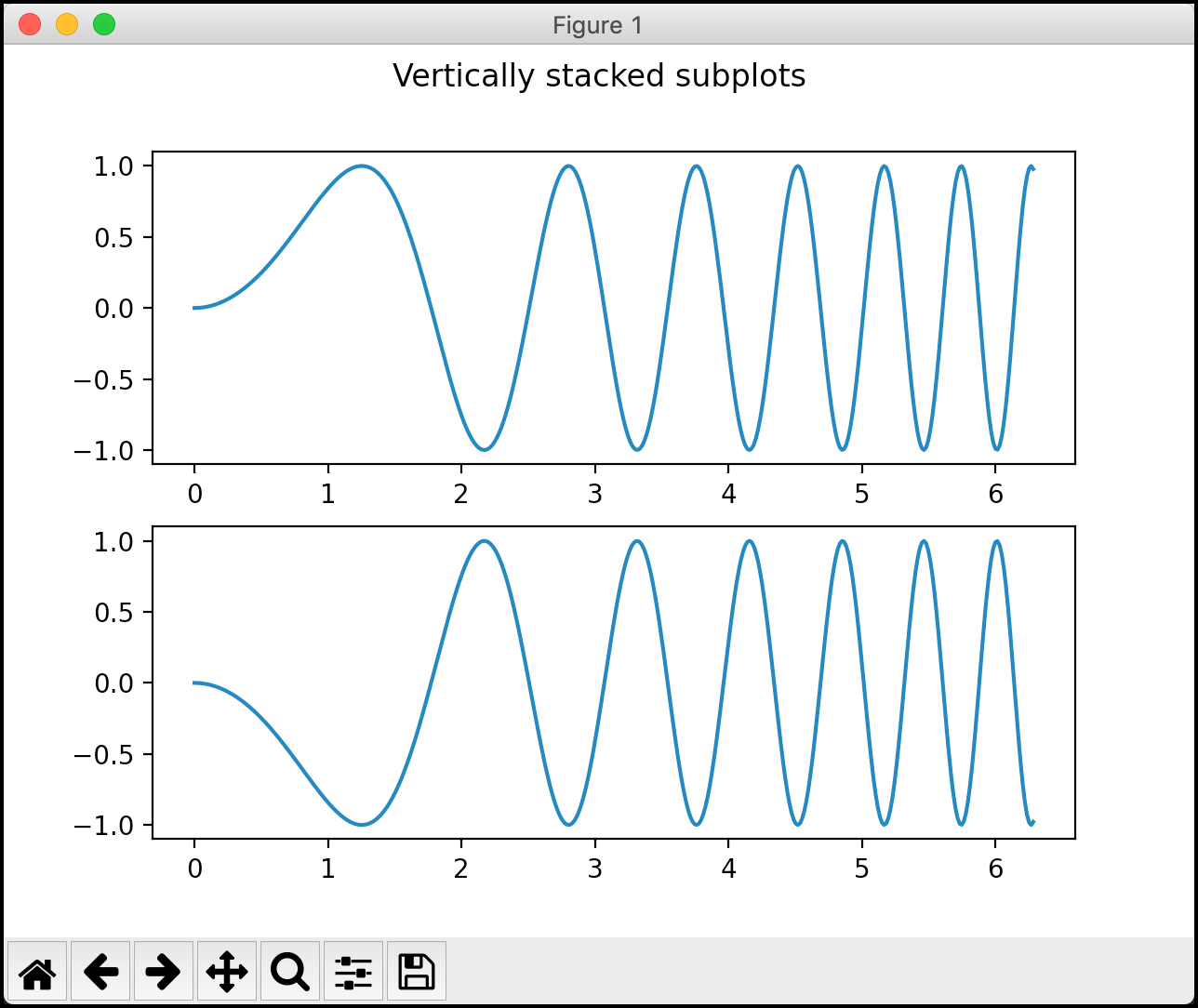If you don’t want to use NumPy, you could plot the two sets of numbers from the previous example. In fact, you should try that. Go ahead and create a new file named `multiple_plots2.py` and add this code:

```# multiple_plots2.py

import matplotlib.pyplot as plt

def multiple_plots():
numbers = [2, 4, 1, 6]
more_numbers = [5, 1, 10, 3]
fig, axs = plt.subplots(2)
fig.suptitle('Vertically stacked subplots')
axs.plot(numbers)
axs.plot(more_numbers)
plt.show()

if __name__ == '__main__':
multiple_plots()
```

In this code, you remove the NumPy code entirely and add the two lists of numbers from the earlier example. Then you plot them using the `Axes` object.

This results in the following stacked plot: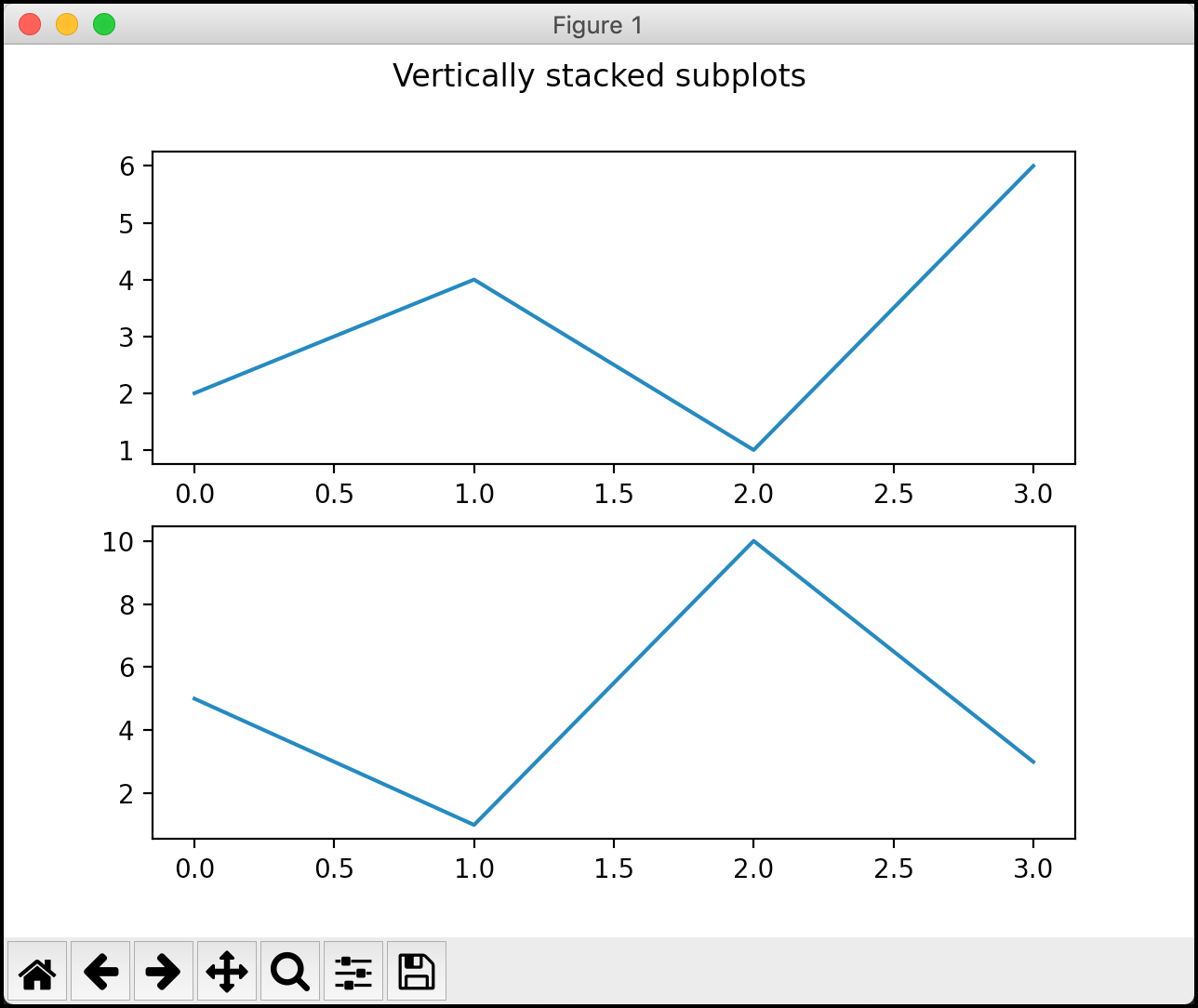At this point, you should have a pretty good handle on how to create multiple figures and stacked plots with Matplotlib.

## Wrapping Up

Matplotlib is a great package that you can use to create all kinds of neat graphs. It’s amazing how few lines of code you need to write to create a useful plot from your data. In this article, you learned about the following topics:

• Creating a Simple Line Chart with PyPlot
• Creating a Bar Chart
• Creating a Pie Chart